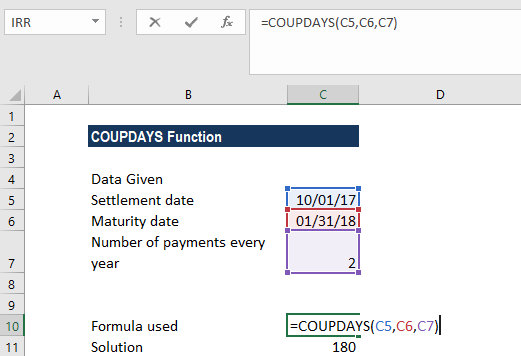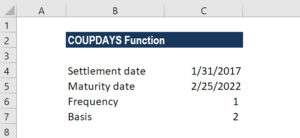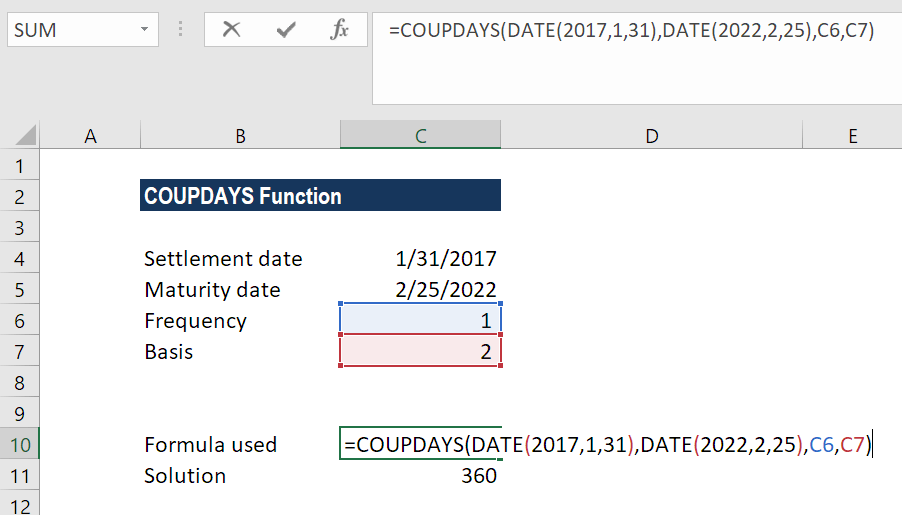# COUPDAYS Function

Calculates the number of days in the coupon period that contains the settlement date

## What is the COUPDAYS Function?

The COUPDAYS Function is categorized under financial functions. It helps calculate the number of days in the coupon period that contains the settlement date. Coupon bonds are bonds that pay interest before the maturity of the bond.

As a bond purchaser, we will be interested in knowing how long we need to wait until we receive our first interest payment. The COUPDAYS function helps in calculating the number of days between a coupon period’s beginning and settlement date.

### Formula

=COUPDAYS(settlement, maturity, frequency, [basis])

The COUPDAYS function uses the following arguments:

1. Settlement (required argument) – This is the settlement date of a given security. It is the date after the security is traded to the buyer.
2. Maturity (required argument) – This is the date when the security expires.
3. Frequency (required argument) – The number of coupon payments per year. The argument can take a value of 1 (annual payment), 2 (semi-annual payments), or 4 (quarterly payments).
4. Basis (optional argument) – It specifies the day count basis to be used. It uses one of the following values:

BasisDay Count basis
0 or omittedUS(NASD) 30/360
1Actual/actual
2Actual/360
3Actual/365
4European 30/360

The function will default to 0 when omitted. It indicates that the days in the month are counted using the US 30-day method with a 360-day year. When we enter 1 as the basis, the function uses the actual number of days in the month and year. Whereas, when we enter 2, it will count the actual days in the month with a 360-day year, while 3 will assume a 365-day year. When we enter 4 as the basis, it is the same as 1 except that it uses the European 30-day method.

### How to use COUPDAYS Function in Excel?

To understand the uses of the COUPDAYS function, let’s consider a few examples:

#### Example 1

Let’s see how the COUPDAYS function works when are given the following data:Additionally, we are told that 2 payments are made per year.

Using the formula =COUPDAYS(C5,C6,C7), we get the result of 180. We left the basis as 0 so the function used the US 30/360 as count days.#### Example 2

As the COUPDAYS function doesn’t accept dates in text format, we need to convert them into a DATE format. Let’s see an example to understand it. Suppose we are given the following data:As we need to find the number of days using the function, we need to first convert the dates given into text format.

The formula to be used would be =COUPDAYS(DATE(2017,1,31),DATE(2022,2,25),1,2).

The result we get here is 360. Excel first converted the dates in text format into proper dates and then calculated the number of days.### Few notes about the COUPDAYS Function

1. #NUM! error – Occurs in the following scenarios:
• When the settlement date provided is greater than or equal to (≥) the maturity date.
• When the given frequency argument provided by the user is not equal to 1, 2 or 4.
• When the given basis argument is a number other than 0, 1, 2, 3 or 4.
2. #VALUE! error – Occurs in the following scenarios:
• When the given settlement date or maturity date is not a valid date. Remember that we need to enter dates in date format or else use the DATE function to convert them into a proper date format. The function doesn’t work when dates given are in text format. For example, =COUPDAYS(“1/25/2023″,”11/15/2024”,2,1) = #VALUE.
• Any of the arguments given are non-numeric.
3. The COUPDAYS function truncates all arguments to integers.

Thanks for reading CFI’s guide to the COUPDAYS Excel function. By taking the time to learn and master Excel functions, you’ll significantly speed up your financial analysis. To learn more, check out these additional CFI resources:

• Excel Functions for Finance
• Advanced Excel Formulas Course
• Advanced Excel Formulas You Must Know
• Excel Shortcuts for PC and Mac

### Free Excel Tutorial

To master the art of Excel, check out CFI’s FREE Excel Crash Course, which teaches you how to become an Excel power user.  Learn the most important formulas, functions, and shortcuts to become confident in your financial analysis.

Launch CFI’s Free Excel Course now to take your career to the next level and move up the ladder!